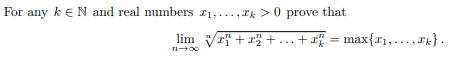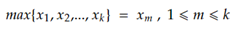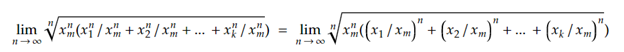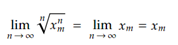# Subtleties in a Radical Limit Problem

#### (A new question of the week)

I find it interesting to observe the process of problem-solving, particularly for proofs: how we discover a solution initially, and then how we turn that into a final answer. Sometimes we can see the main idea in a flash, but the process of writing it as a formal proof reveals subtle issues we didn’t initially notice. This is part of the reason for the importance of proofs in mathematics. The problem we’ll look at here, a limit involving the nth root of a sum, is an interesting illustration of this.

The question came from Gabriel in late January:

Hello, I would like to have a hint on where to begin with solving this problem. Thanks in advance!This is different from the usual limit problems, both in the presence of an unspecified number of unspecified constants, and in the presence of a maximum in the answer. It’s helpful that we don’t have to work out what the limit is (though the thinking I’ll suggest would lead at least to a good guess); most likely we are given the answer precisely because this is unlike other examples that have been shown, and “uses different mental muscles” than most. We have to think creatively!

I was happy that Gabriel wanted only a hint, because that is just how we like to work. I answered, showing my initial thinking without having actually carried it out:

Hi, Gabriel.

I will give you a minimal hint, so you can discover as much as possible yourself.

When I saw this, I first pondered why it might be true, by just imagining how the function (of n) would behave in a simple case, say with k = 2. Why would the maximum determine the limit?

Second, because of the maximum, the word “dominate” came to mind, and the behavior of rational functions. There is a technique that works well for rational limits that can be applied (though quite differently) here.

Please let us know any ideas you have (whether triggered by my ideas, or elsewhere) and show us anything you have tried, so we can see if you need more guidance.

My first thought was to imagine the problem in a specific case with small k and specific numbers $$x_i$$, such as, say,

$$\lim_{n\to\infty}\sqrt[n]{3^n+5^n}$$

(This is a classic Pólya technique: “Consider special cases.” “Solve a simpler problem.”)

What happens as n increases? $$3^n$$ increases fast, and $$5^n$$ increases even faster. For instance, by the time we get to $$n=10$$, we have $$\sqrt{3^{10}+5^{10}}=\sqrt{59,049+9,765,625}$$. Clearly, the second term will leave the first in the dust, so we can practically ignore the first. We say that the second term dominates the first, which becomes negligible; in the limit, we’ll just be taking the nth root of the nth power of 5, which of course will be 5. So the answer makes sense in this simple case, and we can see how the idea will generalize.

How do we turn this idea into a proof? That idea of domination is used in limits involving polynomials, which are dominated by their leading term (the term with the highest power, rather than the largest base as in our problem). A way to deal with that is to divide by the leading term. For example, if we want to find $$\lim_{x\to\infty}\frac{3x^2-5x+4}{4x^2+3x-5},$$ we can divide the numerator and denominator by the leading power, $$x^2$$: $$\lim_{x\to\infty}\frac{3x^2-5x+4}{4x^2+3x-5}=\lim_{x\to\infty}\frac{\frac{3x^2}{x^2}-\frac{5x}{x^2}+\frac{4}{x^2}}{\frac{4x^2}{x^2}+\frac{3x}{x^2}-\frac{5}{x^2}}\\=\lim_{x\to\infty}\frac{3-\frac{5}{x}+\frac{4}{x^2}}{4+\frac{3}{x}-\frac{5}{x^2}}=\frac{3}{4}$$ because all the remaining fractions approach zero in the limit.

I suspected that Gabriel might have seen such problems, and be able to take it from there. I was right.

Gabriel replied,

Hi, Dave. Thanks a lot for the answer, I think I’ve managed to solve it. This was my idea:First I multiplied every element inside the root by (maximumn)/(maximumn).Then I get a bunch of elements smaller than 1 to the power of infinity, which goes to 0. And all that’s left is xmDid I forget something or is it right?

He has used the method I had in mind: He named the maximum, calling its index m, and factored the nth power of that term out of the sum, equivalent to dividing by it. All the terms but one approach 0, and that one becomes $$x_m^n$$.

### But wait! There’s more!

I approved … but then looked again:

Excellent; you got the idea I was hinting at. And it’s nicely formulated.

You might want to explicitly indicate that one of the terms is (xm/xm)n = 1. (A formal proof doesn’t normally include the phrase “a bunch of” 😉 .)

Another way to say this would be that, without loss of generality, you can assume 0 < x1 ≤ x2 ≤ … ≤ xk-1 ≤ xk, so that the maximum is xk, and so on. That is, we can renumber the constants so that we know which is the maximum.

On second thought … putting it this way makes me aware of the fact that there might be two or more of the numbers that are all equal to the maximum. (It doesn’t say distinct real numbers.) Have you covered that possibility?

For example, what if my small case at the start had been $$\lim_{n\to\infty}\sqrt[n]{5^n+5^n}?$$ Then both $$x_1$$ and $$x_2$$ would be the maximum, and there would be two terms that don’t go to zero. Here is what would happen:

$$\lim_{n\to\infty}\sqrt[n]{5^n+5^n}=\lim_{n\to\infty}\sqrt[n]{2\cdot5^n}=\lim_{n\to\infty}5\sqrt[n]{2}=5$$ because the nth root of the constant approaches 1. So it still works; and this example suggests how to handle it.

I wrote it a little informal but just to show my idea. In a test I would definitely try to be more formal 🙂 .

And yes, I thought about the possibility of more values equal to the maximum but I decided not to consider it.

In this case we would get l(1/2).xm with l = number of terms equal xm, right?

Not quite; this is what I had momentarily thought, too, before I wrote anything down! But then, 14 minutes later (while I was still asleep), he wrote again:

Correction:

We wouldn’t get a square root, but l(1/n).xm , and that term goes to 1 for n going to infinity. So it actually wouldn’t matter how many terms are equal xm. The limit will always be equal xm.

I responded:

Good! Again, you’ve seen the implication of my comment, and how to correct for the omission.

What I find interesting here is that one can get the general idea of a proof, from an informal perspective, but not see some important features until we try writing the careful, formal proof. Truth can be a little devious sometimes. (And that’s why I am often a little hesitant to declare something true without doing all the work.)

There could have been a different answer in this special case (if we didn’t trust the statement of the problem – and really, we shouldn’t!). What’s “obviously” true in general is not always true in special cases, so we need to be careful.

So let’s try stating the proof formally:

Without loss of generality, we can assume that $$0<x_1\le x_2\le\dots\lt x_{k-m+1}=\dots=x_{k-1}=x_k$$, so that the last m variables, $$x_{k-m+1},\dots,x_{k-1},x_k$$, are all equal to the maximum, $$x_n$$. Then …

That’s a little ugly. How about if we reverse the numbering to make it easier to describe:

Without loss of generality, we can assume that $$0<x_k\le x_{k-1}\le\dots\lt x_{m}=\dots=x_2=x_1$$, so that the m variables $$x_1,x_2,\dots,x_m$$ are all equal to the maximum, $$x_1$$.

Then for $$m+1\le i\le k$$, $$\lim_{n\to\infty}\left(\frac{x_i}{x_1}\right)^n=0,$$ and for $$1\le i\le m$$, $$\lim_{n\to\infty}\left(\frac{x_i}{x_1}\right)^n=\lim_{n\to\infty}\left(\frac{x_1}{x_1}\right)^n=1.$$

Consequently, $$\lim_{n\to\infty}\sqrt[n]{x_1^n+x_2^n+\dots x_k^2}=\\\lim_{n\to\infty}\sqrt[n]{x_1^n\left(\left(\frac{x_1}{x_1}\right)^n+\dots+\left(\frac{x_m}{x_1}\right)^n+\left(\frac{x_{m+1}}{x_1}\right)^n+\dots+\left(\frac{x_k}{x_1}\right)^n\right)}=\\\lim_{n\to\infty}x_1\cdot\sqrt[n]{\left(\frac{x_1}{x_1}\right)^n+\dots+\left(\frac{x_m}{x_1}\right)^n+\left(\frac{x_{m+1}}{x_1}\right)^n+\dots+\left(\frac{x_k}{x_1}\right)^n}=\\x_1\cdot\lim_{n\to\infty}\sqrt[n]{\underset{m}{\underbrace{1+\dots+1}}+0+\dots+0}=\\x_1\cdot\lim_{n\to\infty}\sqrt[n]{m}=x_1\cdot1=\max(x_1,\dots x_k)$$

This site uses Akismet to reduce spam. Learn how your comment data is processed.